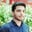Related Tags

objective c

# NSNumbers and their types in Objective-CSalman Yousaf

### Overview

Objective-C offers a wide range of functions regarding NNumber for saving the basic data types such as float, int, and so on. There is a wide range of functions provided by Objective-C that work with NSNumber.

### Syntax

Syntax to create an object of NSNumber type:

+(NSNumber*) variableName: (Type) value

Syntax to create an architecture-independent data type value:

DataType variableName

### Create NSNumber Objects

Some important methods to create variables of NSNumber are as follows:

(NSNumber*) numberWithChar: (char)value

It helps to create and return an NSNumber object. This object contains the given value and treats it as a signed character:

(NSNumber*) numberWithBool:(BOOL)value

It helps to create and return an NSNumber object. This object contains the given value and treats it as bool:

(NSNumber*) numberWithDouble:(double)value

It helps to create and return an NSNumber object. This object contains the given value and treats it as double:

(NSNumber*) numberWithFloat:(float)value

It helps to create and return an NSNumber object. This object contains the given value and treats it as the float:

(NSNumber*) numberWithInt:(int)value

It helps to create and return an NSNumber object. This object contains the given value and treats it as signed integer:

(NSNumber*) numberWithInteger:(NSInteger)value

It helps to create and return an NSNumber object. This object contains the given value and treats it as NSInteger.

### Create language data type variables

(float) floatValue

It returns the specified value as a float.

(double) doubleValue

It returns a specified value as double.-(char)charValue. It returns a specified value as a character.

(BOOL) boolValue

It returns the specified value as a bool.

(int)intValue

It returns the specified value as an integer type.

(NSInteger) integerValue

It returns the specified value in the form of an NSInteger.

(NSString) stringValue

It returns the specified value in the form of a human-readable string.

### Example

In this example, we show how to create and initialize language defined as well as NSNumber type objects:

#import <Foundation/Foundation.h>
/*it will inherit an interface to the runtime system */
@interface demoClass: NSObject
- (NSNumber *) addc1:(NSNumber *)c1 withc2:(NSNumber *)c2;
@end
/* implementation of of above declared method*/
@implementation demoClass
- (NSNumber *) addc1:(NSNumber *) c1 withc2:(NSNumber *) c2 {
float n1 = [c1 floatValue];
float n2 = [c2 floatValue];
float sum = n1 + n2;
NSNumber *ans = [NSNumber numberWithFloat: sum];
return ans;
}

@end
/* main to check above class method*/
int main() {
NSAutoreleasePool * pool = [[NSAutoreleasePool alloc] init];
/* test above created method*/
demoClass *tclass = [[demoClass alloc] init];
NSNumber *c1 = [NSNumber numberWithFloat: 22.9];
NSNumber *c2 = [NSNumber numberWithFloat: 18.0];
NSNumber *ans = [tclass addc1:c1 withc2: c2];
NSString *ansstring = [ans stringValue];
NSLog(@"The sum is %@", ansstring);
[pool drain];
return 0;
}
Objective-c function for calculating sum

### Explanation

• Line 3: NSObject is a root class demoClass, which allows it to behave like an objective-C object. The demoClass class inherits an interface to the runtime system. It also contains a method to add two numbers.
• Lines 7–13: @implementation demoClass contains actual code implementation of the declared method above to add two values.
• Line 8: We add two values, c1 and c2.
• Lines 9–10: We declare and define two float values as copies of c1 and c2.
• Line 11: We define sum to store arithmetic sum results.
• Line 12: We declare ans to convert into an NSNumber object from a floating-point value.
• Line 13: We return the value as an NSNumber object.
• Lines 18–26: The main function to check the above program.
• Lines 19–27: NSAutoreleasePool *pool will allocate a program memory pool while [pool drain] will drain the pool to release memory.
• Line 21: We create an instance of demoClass.
• Lines 22–23: We create two objects of NSNumber with default values 22.9 and 18.0, respectively.
• Line 24: We invoke the addc1 withc2 method to calculate arithmetic addition.
• Lines 25–26: We get the addition of two numbers, c1 and c2. We print results to the console.

RELATED TAGS

objective c

CONTRIBUTORSalman Yousaf
RELATED COURSES

View all Courses

Keep Exploring

Learn in-demand tech skills in half the time